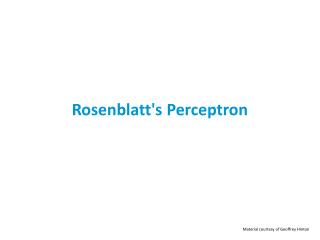# Rosenblatt's Perceptron - PowerPoint PPT PresentationDownload PresentationRosenblatt's Perceptron

Rosenblatt's Perceptron
Download Presentation## Rosenblatt's Perceptron

- - - - - - - - - - - - - - - - - - - - - - - - - - - E N D - - - - - - - - - - - - - - - - - - - - - - - - - - -
##### Presentation Transcript

1. Rosenblatt's Perceptron Material courtesy of Geoffrey Hinton

2. The history of perceptrons • Invented by the psychologist Frank Rosenblatt in 1958. • The first successful algorithm for training neurons. • Still widely used today for tasks with enormous feature vectors that contain many millions of features.

3. The standard paradigm for statistical pattern recognition The standard Perceptron architecture decision unit • Convert the raw input vector into a vector of feature activations. • Learn how to weight each of the feature activations to get a single scalar quantity. • If this quantity is above some threshold, decide that the input vector is a positive example of the target class. learned weights feature units

4. Binary threshold neurons (decision units) • McCulloch-Pitts (1943) • First compute a weighted sum of the inputs from other neurons. • Then output a 1 if the weighted sum exceeds zero. 1 1 if y 0 0 otherwise z 0

5. The bias term • A threshold is equivalent to having a negative bias. • We can avoid having to figure out a separate learning rule for the bias by using a trick: • A bias is exactly equivalent to a weight on an extra input line that always has an activity of 1. • We can now learn a bias as if it were a weight.

6. The perceptron convergence procedure: Training binary output neurons as classifiers • Pick training cases using any policy that ensures that every training case will keep getting picked. • If the output unit is correct, leave its weights alone. • If the output unit incorrectly outputs a zero, add the input vector (multiplied by the learning rate) to the weight vector. • If the output unit incorrectly outputs a 1, subtract the input vector (multiplied by the learning rate) from the weight vector. • This is guaranteed to find a set of weights that gets the right answer for all the training cases if any such set exists.

7. Example

8. Weight-space • This space has one dimension per weight. • A point in the space represents a particular setting of all the weights. • Assuming that we have eliminated the threshold, each training case can be represented as a hyperplane through the origin. • The weights must lie on one side of this hyper-plane to get the answer correct.

9. Each training case defines a plane(shown as a black line) The plane goes through the origin and is perpendicular to the input vector. On one side of the plane the output is wrongbecause the scalar product of the weight vector with the input vector has the wrong sign. Weight space an input vector with correct answer=1 good weight vector right side wrong side o the origin bad weight vector

10. Each training case defines a plane(shown as a black line) The plane goes through the origin and is perpendicular to the input vector. On one side of the plane the output is wrongbecause the scalar product of the weight vector with the input vector has the wrong sign. Weight space wrong side right side bad weights an input vector with correct answer=0 good weights o the origin

11. The cone of feasible solutions an input vector with correct answer=0 • To get all training cases right we need to find a point on the right side of all the planes. • There may not be any such point! • If there are any weight vectors that get the right answer for all cases, they lie in a hyper-cone with its apex at the origin. • So the average of two good weight vectors is a good weight vector. • The problem is convex. wrong right bad weights good weights right wrong an input vector with correct answer=1 o the origin

12. Why the learning procedure works(first attempt) • Consider the squared distance between any feasible weight vector and the current weight vector. • Hopeful claim: Every time the perceptron makes a mistake, the learning algorithm moves the current weight vector closer to all feasible weight vectors. feasible right wrong Problem case: The weight vector may not get closer to this feasible vector! current

13. Why the learning procedure works • So consider “generously feasible”weight vectors that lie within the feasible region by a margin at least as great as the length of the input vector that defines each constraint plane. • Every time the perceptron makes a mistake, the squared distance to all of these generously feasible weight vectors is always decreased by at least the squared length of the update vector. • If a feasible weight vector exist, then a “generously feasible”also exist. margin right wrong

14. Informal sketch of proof of convergence • Each time the perceptron makes a mistake, the current weight vector moves to decrease its squared distance from every weight vector in the “generously feasible” region. • The squared distance decreases by at least the squared length of the input vector. • So after a finite number of mistakes, the weight vector must lie in the feasible region if this region exists.